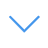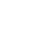# Geometry Fresh

Geometry Fresh is one of the Math Games that you can play on UGameZone.com for free. Exercise your math skills with this game! Can you see the pattern of the game? Find the corresponding figure of the number using the mathematical algorithm calculation, choose the correct answer!

Read Moreاضغط على 【Esc】 للخروج من وضع ملء الشاشة

# Geometry Fresh

Geometry Fresh is one of the Math Games that you can play on UGameZone.com for free. Exercise your math skills with this game! Can you see the pattern of the game? Find the corresponding figure of the number using the mathematical algorithm calculation, choose the correct answer!لتشغيل اللعبة ، تحتاج إلى السماح بتشغيل Adobe Flash Player في متصفحك.

You can use UGameZone Flash Enabler plugin to enable Flash automatically. Or you can follow our instructions to enable Flash manually.

play automatically

## Game Ratings

86%

يلعب 1490

تقييم 86%

الأصوات 781

781
162
Category
Math Games
الكلمات
وصف

Geometry Fresh is one of the Math Games that you can play on UGameZone.com for free. Exercise your math skills with this game! Can you see the pattern of the game? Find the corresponding figure of the number using the mathematical algorithm calculation, choose the correct answer!

Step 1
Step 2